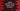# Python program to generate secure random string of length n## Introduction :

This tutorial will show you how to generate one secured random string of specific length in python. In most cases, we need to create a random string. For example, if you are storing some information and you need one key for that, you can create one random string and use it.

Note that, even though we are generating one random string in this example, there is a slight possibility that the program will generate one same string on two different execution. So, if you want to generate random strings for your production server, make sure to add extra layer of calculation like adding the current server date/time etc. to make it more unique.

## Python way to generate a random string :

To get a random string, we need to pick one random character from all the characters that we have. For that, we will use two constant fields defined in python string class :

• ascii_letters : It is the combination of all ASCII lowercase and upper case letters. If you want only upper case or lower case letters, you can use ascii_lowercase or ascii_uppercase.
• digits: It is the string 0123456789

So, we can generate the random string by randomly picking characters from ascii_letters and digits.

## What method to use:

Python provides one module called random for random data generation. To get one random character from a string, we will use the below method :

``random.choice(sequence)``

If we pass one string or any sequence to this method, it will return one random character.

### Get secure random values in python 3.6:

For python 3.6 and above, you can use another module called secrets. This is used to generate cryptographically strong random values those are useful for any secure operations.

It also provides the same choice method :

``secrets.choice(sequence)``

In this example, we will use this method.

## Python program :

Let me show you the python program :

``````import secrets
import string

char_string = string.ascii_letters + string.digits

def getRandomString(size):
return ''.join(secrets.choice(char_string) for _ in range(size))

print(getRandomString(4))
print(getRandomString(10))
print(getRandomString(24))``````

Here, we are using join to join all random characters that we have created using a for loop and secrets.choice.

It will create one output as like below :

``````DETX
M0OGExhxee
2afe6iy6VG6ZjtlygjYxlqK8``````